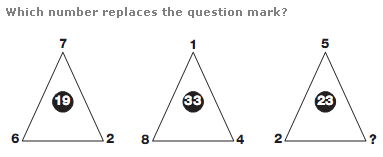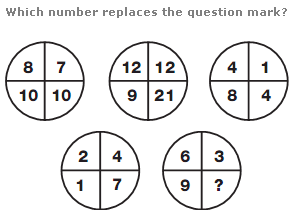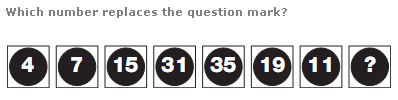# Puzzles - Number puzzles

Exercise : Number puzzles
277.:
9
Explanation
:
In each triangle, multiply the lower two numbers together and add the upper number to give the value in the centre.

278.:
3
Explanation
:
Using the lower two circles as a source, the values in corresponding segments of the upper left circle equal the sums of the numbers in the lower two circles. The values in the upper central circle equal the products of the values in the lower two circles, and the upper right circle equals the difference between values in the lower two circles.

279.